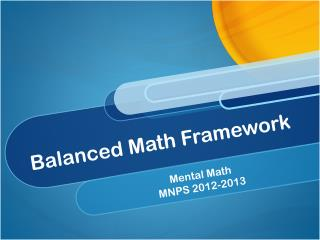DownloadDownload PresentationBalanced Math Framework

# Balanced Math Framework

Download Presentation## Balanced Math Framework

- - - - - - - - - - - - - - - - - - - - - - - - - - - E N D - - - - - - - - - - - - - - - - - - - - - - - - - - -
##### Presentation Transcript

1. Balanced Math Framework Mental Math MNPS 2012-2013

2. Elements of Balanced Math

3. Common CoreandBalanced Math

4. What’s In? • Student Centered • Conceptual Understanding • Problem Solving • What’s Out? • Traditional , textbook driven • Teacher Centered • Rote Learning without Meaning

5. Conceptual Understanding Anatomy of a Lesson Skill Acquistion

6. How do I use my math time 60 minute of math daily !

7. Mental Math

8. CCSS Required Fluencies • K Add/subtract within 5 • 1 Add/subtract within 10 • 2 Add/subtract within 20. Add/subtract within 100 (pencil and paper) . By end of year, know from memory all sums of two one‐digit numbers • 3 Multiply/divide within 100. Add/subtract within 1000. By end of year, know from memory all products of two one‐digit numbers • 4 Add/subtract within 1,000,000

9. Memorization vs Automaticity • Memorization of basic facts usually refers to committing the results of unrelated operations to memory so that thinking through a computation is unnecessary. • Isolated additions and subtractions are practiced one after another as if there were no relationships among them; the emphasis is on recalling the answers. • Teaching facts for automaticity, in contrast, relies on thinking. Answers to facts must be automatic, produced in only a few seconds; counting each time to obtain an answer is not acceptable. But thinking about the relationships among the facts is critical. • The issue here is not whether facts should eventually be memorized but how this memorization is achieved: by drill and practice, or by focusing on relationships.

10. Memorization vs Automaticity • Isn’t memorization faster? Interestingly, no! • When relationships are the focus, there are far fewer facts to remember, and big ideas like compensation and part-whole relationships come into play. Also, a child who forgets an answer has a quick way to calculate it. • Children who commit the facts to memory easily are able to do so because they have constructed relationships among the facts.

11. Have you practiced making combinations of five and ten?

12. You are getting faster at this! Keep practicing!

13. Memorization vs Automaticity • Double plus or minus—for example, 6 + 7= 6 +6 + 1 (or 7 +7 – 1) =13. • Working with the structure of five—for example, 6 + 7 = 5 + 1 + 5 + 2 =10 + 3 =13. • Making ten—for example, 9 + 7 =10 + 6 =16. • Using compensation—for example, 6 + 8 = 7 + 7 = 14. • Using known facts—for example, 6 + 8 = 14, so 7 + 8 must be 14 + 1 =15.

14. Number Wars • Ace = 1; Face cards= 10 • Put a card down and first to call out answer. Put card down before looking at it; Think Mentally; Penalty is 5 cards • Partner A and Partner B • (Partner A) + (Partner B) • Write down the 5 most difficult facts and use as a reference sheet • (Partner A) x (Partner B) • (Double Partner A) + (Partner B)

15. Vocabulary beyond the Word Wall • First even whole number • First prime number • First 2-digit prime number • How do you cube a number • Faces on a cube • Degrees in a right angle • Minutes in an hour

16. Mental String • Start with number of faces on a cube • Multiply by the first even prime number • Add the digits • Cube the results • Round to the nearest 10 • Add the number of degrees in a right angle • Divide your answer by the number of minutes in an hour

17. Mental String • Start with number of faces on a cube- 6 • Multiply by the first even prime number- 6 x 2=12 • Add the digits- 1+2=3 • Cube the results- 3x3x3=27 • Round to the nearest 10 - 30 • Add the number of degrees in a right angle- 30 + 90=120 • Divide your answer by the number of minutes in an hour- 120/60= 2

18. Mental Strings • Create strings for grade level • Preview vocabulary • Congratulate success • Allow one student to model • Invite students to write their own

19. Mental Strings • Sides on a triangle • First even number • First odd number • Quarts in a gallon • Double a number

20. Mental Strings • Start with two more than a number of sides on a triangle • Multiply by itself • Add the first odd whole number • Add the digits of your answer together • Add the number of quarts in a gallon • Double your number

21. Mental Strings • Start with two more than a number of sides on a triangle------5 • Multiply by itself ------5 x5=25 • Add the first odd whole number------25 + 1=26 • Add the digits of your answer together----2 +6=8 • Add the number of quarts in a gallon----8 +4=12 • Double your number 12 x 2= 24

22. Mental Math String – 3rd Grade • Start with number of sides in a triangle • Double your answer • Add the number of diagonals that can be drawn on a square • Multiply by the number of sides on a pentagon

23. QUESTIONS?